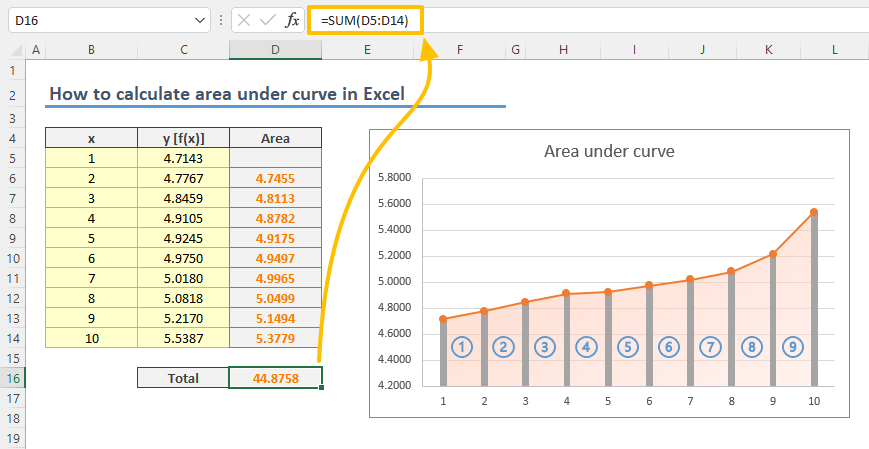In this guide, we’re going to show you how to calculate area under curve in Excel.

Unfortunately, there isn't a single function or feature that can calculate area under curve in Excel. You can still rely on integrals to calculate area. On the other hand, Excel can help us with a simpler approach as well.

## Calculating area under curve by trapezoidal rule

The trapezoidal rule is a technique for approximating the region under the graph as a trapezoid and calculating its area. The rule can be depicted as following:Because we have the data which generates the chart, we can easily transform the above formula into a format that Excel can calculate each trapezoid's area individually.1. Start by inserting a helper column in your data set. Adjacent columns will ease copying of the formula. This column will calculate the area of each trapezoid between data points (x).2. Enter the area formula starting from the second row. The formula will refer the data points in the same (k) and the previous row (k-1).
=(C6+C5)/2*(B6-B5)3. Sum all area values to find the total area under the curve.## Using Chart Trendline

An alternative approach is to use the equation of the plotted curve. Excel can plot a trendline based on your values and generate an equation for the trendline as well.

2. Either use the Chart Elements button (plus button at the top-right) or Add Chart Element command in the Chart Design tab of the Ribbon to select More Options item.3. More Options command adds a trendline and opens the properties pane at the rights side. Under Trendline Options, select Polynomial type and check the Display Equation on chart property.4. Next step is the trickiest of this article if you are not familiar with integrals. Briefly, you need the convert the equation to its definite integral and calculate the minimum and maximum values through the definite integral. The difference between the two results will give the area under curve.
You need to increase the power of each x value by 1 and divide it by the increased power value. For example, x² becomes x³/3. According to this our formula will be the following:5. Next step is to calculate the definite integral values for the smallest and the largest x. You can omit the c values since the subtracting operations nullifies them. These are 1 and 10 in our example:6. Final step is to find the difference to calculate the area under curve.## Remarks

As you can realize there is a small difference between the results of each approach. Since each approach returns an approximate value, it would be better to use both and compare.

If you are a Microsoft 365 subscriber, you can use either the LET or the LAMBDA functions to simplify the use of the definite integral formula multiple times.